• Размер: 227 Кб
• Количество слайдов: 13

## Описание презентации General Heat Engineering Laws of Thermodynamics In по слайдам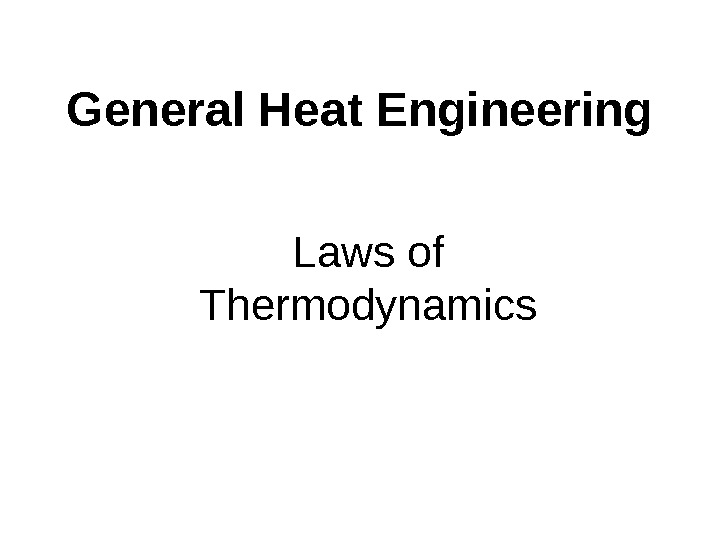General Heat Engineering Laws of Thermodynamics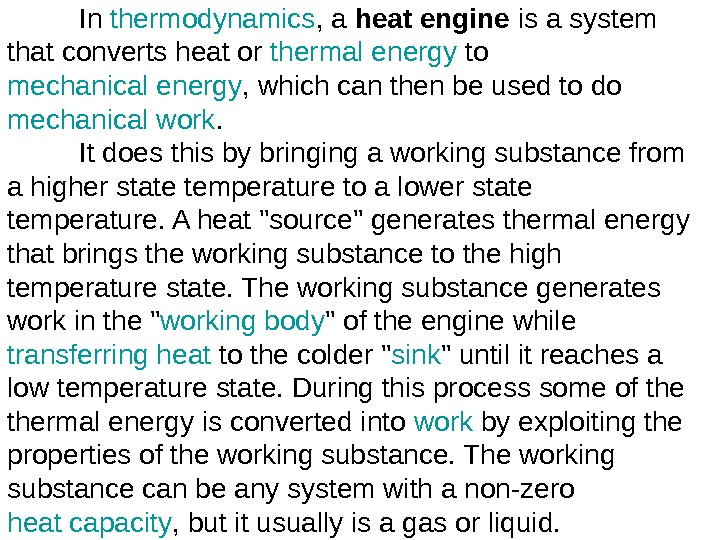In thermodynamics , a heat engine is a system that converts heat or thermal energy to mechanical energy , which can then be used to do mechanical work. It does this by bringing a working substance from a higher state temperature to a lower state temperature. A heat «source» generates thermal energy that brings the working substance to the high temperature state. The working substance generates work in the » working body » of the engine while transferring heat to the colder » sink » until it reaches a low temperature state. During this process some of thermal energy is converted into work by exploiting the properties of the working substance. The working substance can be any system with a non-zero heat capacity , but it usually is a gas or liquid.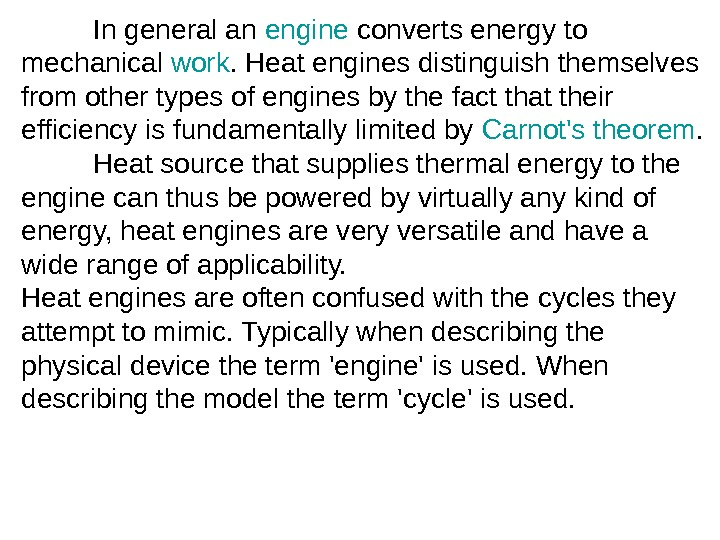In general an engine converts energy to mechanical work. Heat engines distinguish themselves from other types of engines by the fact that their efficiency is fundamentally limited by Carnot’s theorem. H eat source that supplies thermal energy to the engine can thus be powered by virtually any kind of energy, heat engines are very versatile and have a wide range of applicability. Heat engines are often confused with the cycles they attempt to mimic. Typically when describing the physical device the term ‘engine’ is used. When describing the model the term ‘cycle’ is used.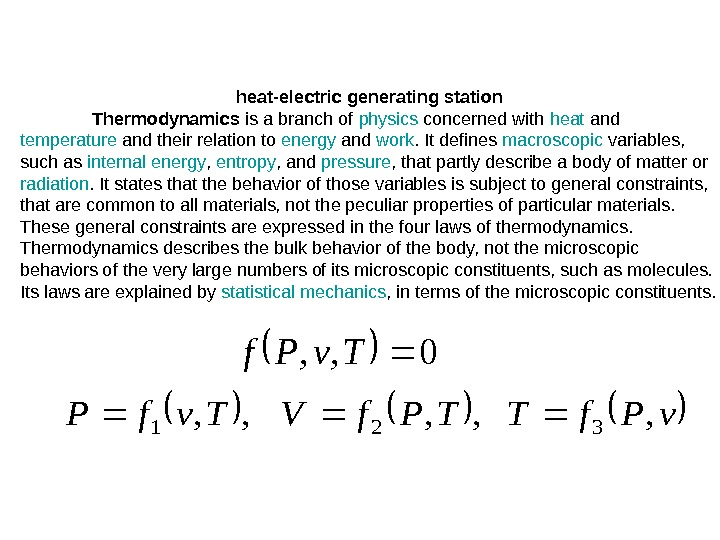heat-electric generating station Thermodynamics is a branch of physics concerned with heat and temperature and their relation to energy and work. It defines macroscopic variables, such as internal energy , entropy , and pressure , that partly describe a body of matter or radiation. It states that the behavior of those variables is subject to general constraints, that are common to all materials, not the peculiar properties of particular materials. These general constraints are expressed in the four laws of thermodynamics. Thermodynamics describes the bulk behavior of the body, not the microscopic behaviors of the very large numbers of its microscopic constituents, such as molecules. Its laws are explained by statistical mechanics , in terms of the microscopic constituents. 0, , Tv. Pf. TTPf. VTvf. P, , ,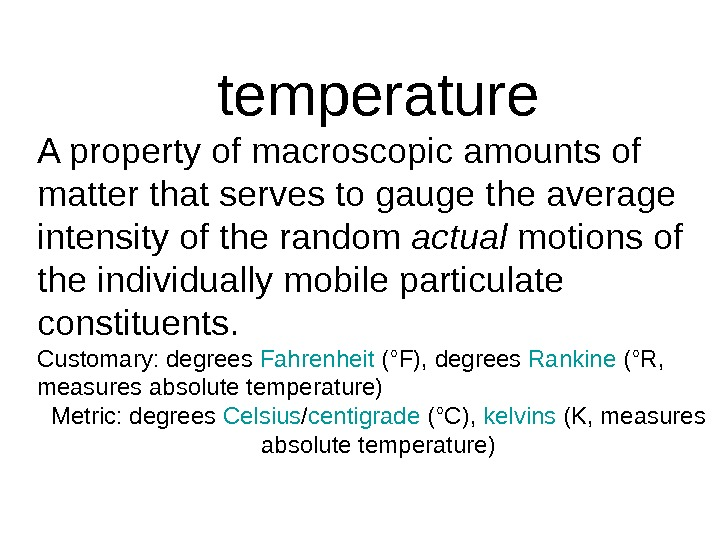temperature A property of macroscopic amounts of matter that serves to gauge the average intensity of the random actual motions of the individually mobile particulate constituents. Customary: degrees Fahrenheit (°F), degrees Rankine (°R, measures absolute temperature) Metric: degrees Celsius / centigrade (°C), kelvins (K, measures absolute temperature)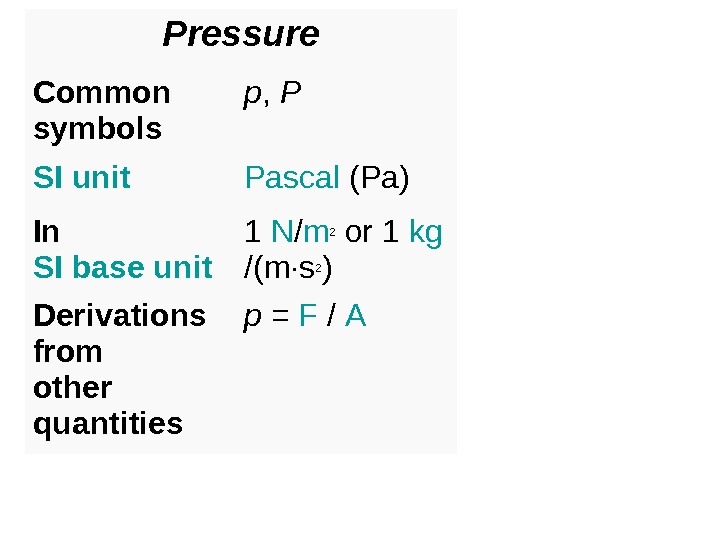Pressure Common symbols p , P SI unit Pascal (Pa) In SI base unit s 1 N / m 2 or 1 kg /( m · s 2 ) Derivations from other quantities p = F /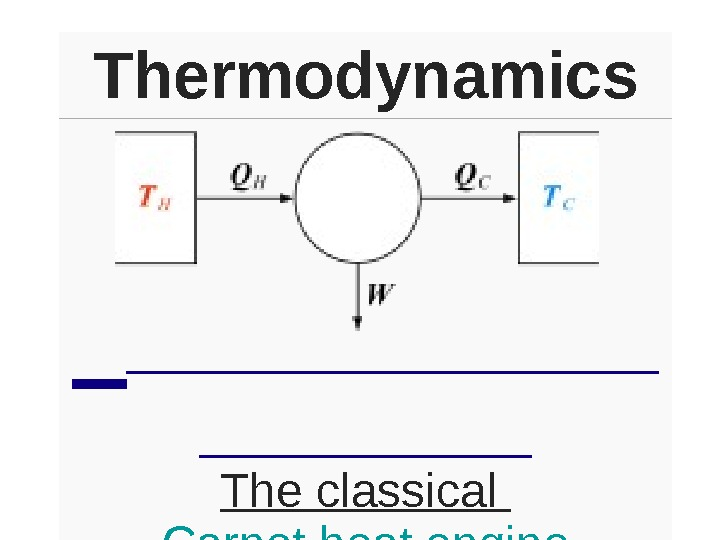Thermodynamics The classical Carnot heat engine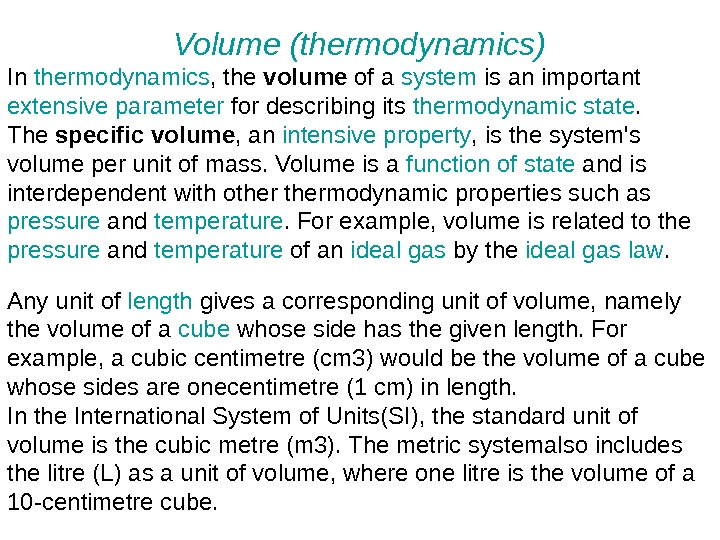Volume (thermodynamics) In thermodynamics , the volume of a system is an important extensive parameter for describing its thermodynamic state. The specific volume , an intensive property , is the system’s volume per unit of mass. Volume is a function of state and is interdependent with othermodynamic properties such as pressure and temperature. For example, volume is related to the pressure and temperature of an ideal gas by the ideal gas law. Any unit of length gives a corresponding unit of volume, namely the volume of a cube whose side has the given length. For example, a cubic centimetre (cm 3) would be the volume of a cube whose sides are onecentimetre (1 cm) in length. In the International System of Units(SI), the standard unit of volume is the cubic metre (m 3). The metric systemalso includes the litre (L) as a unit of volume, where one litre is the volume of a 10 -centimetre cube.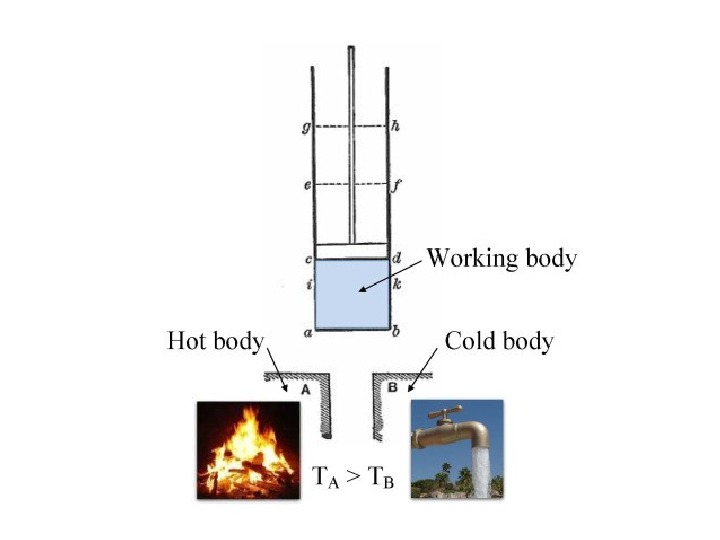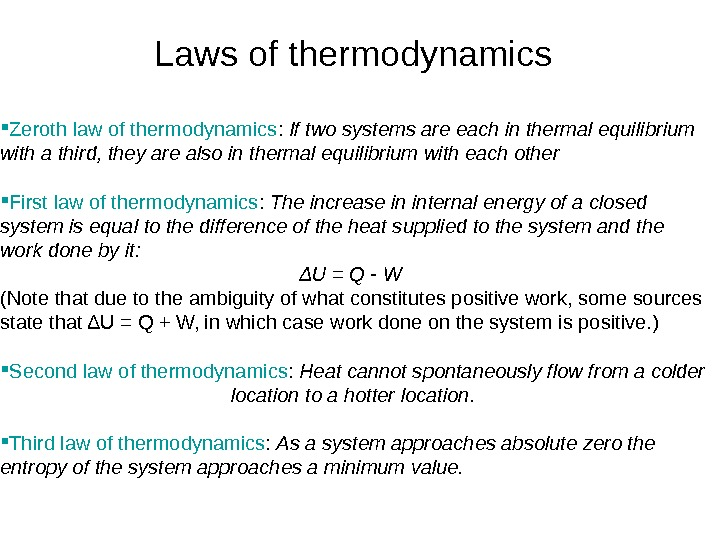Laws of thermodynamics Zeroth law of thermodynamics : If two systems are each in thermal equilibrium with a third, they are also in thermal equilibrium with each other First law of thermodynamics : The increase in internal energy of a closed system is equal to the difference of the heat supplied to the system and the work done by it: ΔU = Q — W (Note that due to the ambiguity of what constitutes positive work, some sources state that ΔU = Q + W, in which case work done on the system is positive. ) Second law of thermodynamics : Heat cannot spontaneously flow from a colder location to a hotter location. Third law of thermodynamics : As a system approaches absolute zero the entropy of the system approaches a minimum value.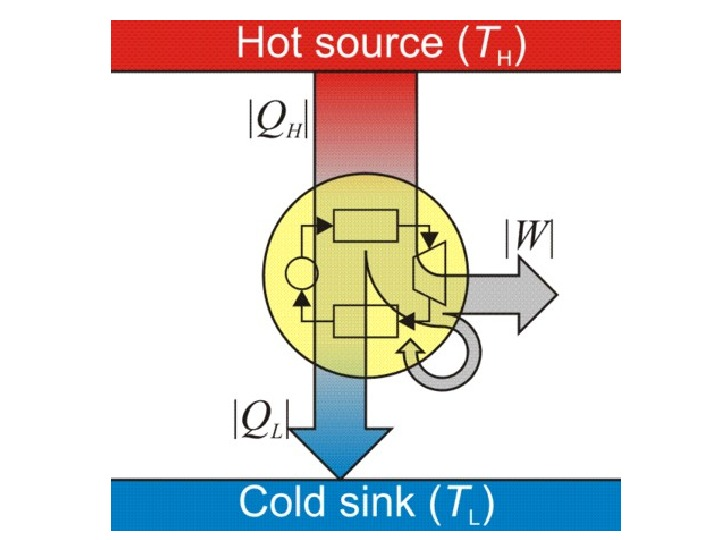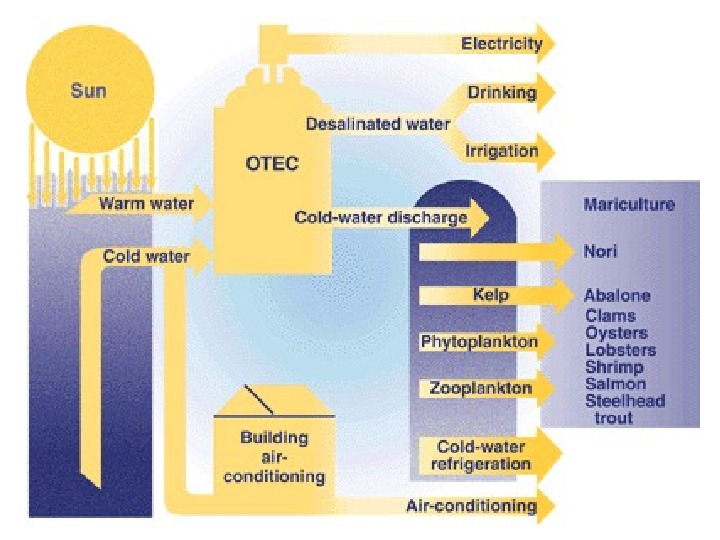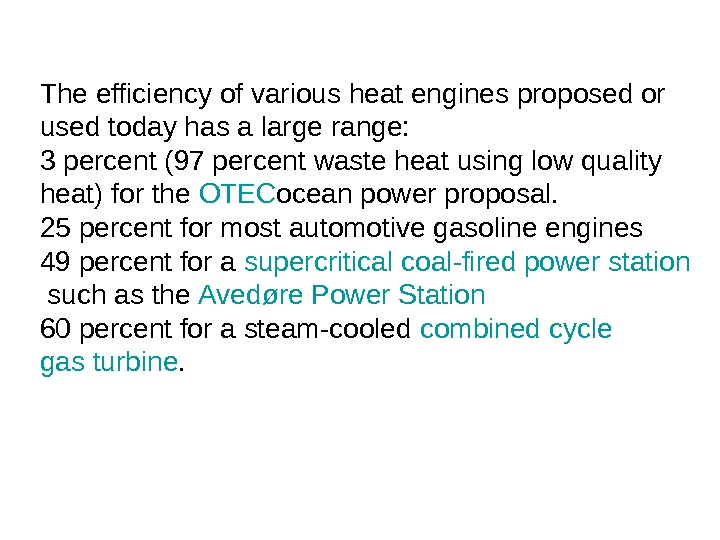The efficiency of various heat engines proposed or used today has a large range: 3 percent (97 percent waste heat using low quality heat) for the OTEC ocean power proposal. 25 percent for most automotive gasoline engines 49 percent for a supercritical coal-fired power station such as the Avedøre Power Station 60 percent for a steam-cooled combined cycle gas turbine.